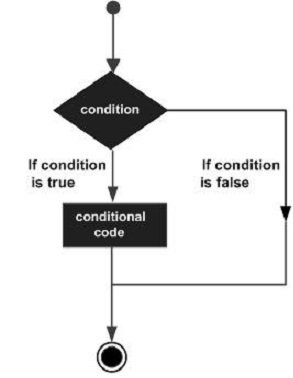# LISP决策LISP提供了以下类型的决策构造。点击以下链接，查看其详细信息。

Construct 描述
cond 这个结构是用于用于检查多个测试行动作条件。它可以嵌套if或其他编程语言语句。
if if结构有多种形式。在最简单的形式，它后面跟着一个测试条，测试操作和一些其它相应措施(次)。如果测试子句的值为true，那么测试的动作被执行，否则，由此产生的子句求值。
when 在最简单的形式，它后面跟着一个测试条和测试操作。如果测试子句的值为true，那么测试的动作被执行，否则，由此产生的子句求值。
case 这种结构实现了像cond 构造多个测试行动语句。但是，它会评估的关键形式，并允许根据该键的形式评价多个行动语句。

## LISP的cond特殊构造

cond的语法是：

```(cond   (test1    action1)
(test2    action2)
...
(testn   actionn))```

```(setq a 10)
(cond ((> a 20)
(format t "~% a is less than 20"))
(t (format t "~% value of a is ~d " a)))```

`value of a is 10`

## if结构

if的语法：

(if (test-clause) (<action1) (action2))

```(setq a 10)
(if (> a 20)
(format t "~% a is less than 20"))
(format t "~% value of a is ~d " a)```

`value of a is 10`

if子句后面可以跟一个可选的then子句：

```(setq a 10)
(if (> a 20)
then (format t "~% a is less than 20"))
(format t "~% value of a is ~d " a)```

```a is less than 20
value of a is 10 ```

```(setq a 100)
(if (> a 20)
(format t "~% a is greater than 20")
(format t "~% a is less than 20"))
(format t "~% value of a is ~d " a)```

```a is greater than 20
value of a is 100  ```

when构造，

when宏的语法：

(when (test-clause) (<action1) )

```(setq a 100)
(when (> a 20)
(format t "~% a is greater than 20"))
(format t "~% value of a is ~d " a)```

```a is greater than 20
value of a is 100 ```

case构造

case结构实现像cond结构多个测试动作语句。但是，它会评估的键形式，并允许根据该键的形式评价多个动作语句。

The template for CASE is:

```(case  (keyform)
((key1)   (action1   action2 ...) )
((key2)   (action1   action2 ...) )
...
((keyn)   (action1   action2 ...) ))```

```(setq day 4)
(case day
(1 (format t "~% Monday"))
(2 (format t "~% Tuesday"))
(3 (format t "~% Wednesday"))
(4 (format t "~% Thursday"))
(5 (format t "~% Friday"))
(6 (format t "~% Saturday"))
(7 (format t "~% Sunday")))```

`Thursday`